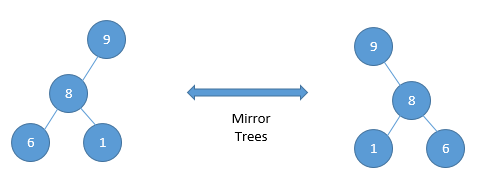Home Trees Given two binary trees, determine if they are mirror trees.

# Given two binary trees, determine if they are mirror trees.

0 comment

Question: Given 2 binary trees, determine if they are mirror of each other

Input: 2 tree pointers are given. (Root pointer)Output: TRUE

We can again apply the recursive sub-structure property of the tree here. The algorithm can be implemented as:-

#include<stdio.h>
#include<malloc.h>

struct binaryTreeNode{
int data;
struct binaryTreeNode * left;
struct binaryTreeNode * right;
};

int areTreeMirrors(struct binaryTreeNode * root1, struct binaryTreeNode * root2)
{
// Terminating condition
if(root1 == NULL && root2 == NULL)
return 1;

//If one of them is NULL, then they are definitely not mirrors
if(root1 == NULL || root2 == NULL)
return 0;

//If one of them is different, then also they are not mirrors
if(root1 -> data != root2 -> data)
{
return 0;
}
else
{
// Compare the left and right nodes of the trees
return (areTreeMirrors(root1 -> left, root2 -> right) && areTreeMirrors(root1 -> right, root2 -> left));
}
}


Time Complexity:- O(n)
Space Complexity:- O(n) due to a recursive stack

#### You may also like

This website uses cookies to improve your experience. We'll assume you're ok with this, but you can opt-out if you wish. Accept Read More